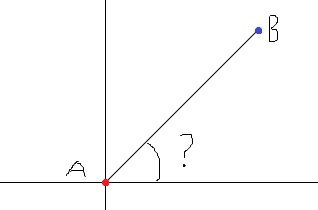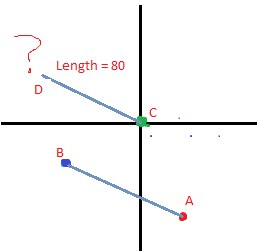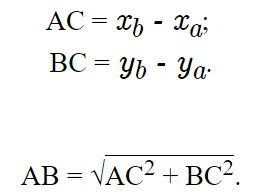## How to find angle? Topic is solved

Get help with using AutoHotkey and its commands and hotkeys
masheen
Posts: 295
Joined: 06 Dec 2016, 14:10

### How to find angle?

Hi forum. How to find angle between two dots?
Can anybody help?i try

Code: Select all

``````ang:=atan2(aX-bX, aY- bY)

MsgBox % ang   ;2.359474

Return dllcall("msvcrt\atan2","Double",y, "Double",x, "CDECL Double")
}
``````
but i get 2.359474 why not 45 ?
wolf_II
Posts: 2688
Joined: 08 Feb 2015, 20:55

### Re: How to find angle?

Angle = ArcTan(dy/dx)
to degrees: Angle = Angle*180/Pi
jeeswg
Posts: 6902
Joined: 19 Dec 2016, 01:58
Location: UK

### Re: How to find angle?  Topic is solved

Code: Select all

``````;tan x = opp / adj

;https://autohotkey.com/docs/commands/Math.htm
;Note: To convert a radians value to degrees, multiply it by 180/pi (approximately 57.29578). To convert a degrees value to radians, multiply it by pi/180 (approximately 0.01745329252). The value of pi (approximately 3.141592653589793) is 4 times the arctangent of 1.

q:: ;calculate angle between 2 points
vAX := 0, vAY := 0
;vBX := 4, vBY := 5
vBX := 4, vBY := 4
MsgBox, % ATan((vBY-vAY)/(vBX-vAX))*57.29578 ;45.000000
MsgBox, % atan2(vBY-vAY, vBX-vAX) ;0.785398
MsgBox, % atan2(vBY-vAY, vBX-vAX)*57.29578 ;45.000000
return

Return dllcall("msvcrt\atan2","Double",y, "Double",x, "CDECL Double")
}
``````
Btw could you post the coordinates for point B? Cheers.

If A is (0,0) and bX=bY, you would expect 0.785398 rad (45 deg) not 2.359474 rad (135.19 deg).
homepage | tutorials | wish list | fun threads | donate
WARNING: copy your posts/messages before hitting Submit as you may lose them due to CAPTCHA
kon
Posts: 1756
Joined: 29 Sep 2013, 17:11

### Re: How to find angle?

masheen
Posts: 295
Joined: 06 Dec 2016, 14:10

### Re: How to find angle?

jeeswg wrote: .
Thx
but now i have new problem

what i do wrong?Code: Select all

``````SearchPointA()
SearchPointB()
SearchPointС()

angle := ATan((bX-aX)/(bY-aY))

length = 80
dX := cX + length * cos(angle)
dY := cY + length * sin(angle)
MouseMove % dX, % dY

ExitApp
``````

oh it is work
but how to do right direction?
jeeswg
Posts: 6902
Joined: 19 Dec 2016, 01:58
Location: UK

### Re: How to find angle?

Btw, do check the order of ATan, it is normally y first, then x.

If other things don't work, you can always do something like:

Code: Select all

``````vDirection := (vDest > vSource) ? 1 : -1
vOffset := Abs(vDistance) * vDirection
``````
homepage | tutorials | wish list | fun threads | donate
WARNING: copy your posts/messages before hitting Submit as you may lose them due to CAPTCHA
masheen
Posts: 295
Joined: 06 Dec 2016, 14:10

### Re: How to find angle?

jeeswg wrote:Btw, do check the order of ATan, it is normally y first, then x.

If other things don't work, you can always do something like:

Code: Select all

``````vDirection := (vDest > vSource) ? 1 : -1
vOffset := Abs(vDistance) * vDirection
``````
Thx

this work wevy good

Code: Select all

``````SearchPointA()
SearchPointB()
SearchPointС()

angle := ATan((bY-aY)/(bX-aX))

length = 180
dX := cX + length * cos(angle) * orderX := aX<bX ? 1: -1
dY := cY + length * sin(angle) *orderX := aX<bX ? 1: -1
MouseMove % dX, % dY
``````
but i have question
can u show me more clear code? or is this ok?
masheen
Posts: 295
Joined: 06 Dec 2016, 14:10

### Re: How to find angle?

Anybody know hot to find the distance from point A to Bcan anybody helm me with code?

distance := sqrt((y2-y1)**2+(X2-X1)**2)

is it right?
kon
Posts: 1756
Joined: 29 Sep 2013, 17:11

### Re: How to find angle?

At the "Compass" link I posted above, search for the function "pxDist".
jeeswg
Posts: 6902
Joined: 19 Dec 2016, 01:58
Location: UK

### Re: How to find angle?

I'm not sure if years of maths study or years of programming will make some of these particular programming challenges any easier, the problem is simply that they are quite fiddly. I believe that my script below is right, all I can do is test it.

Part of the fiddliness is whether the vertical/horizontal distances will be positive/negative as required or whether you have to force it. There may be some trick I'm missing that would make this easier.

Another issue is whether an angle of 30 degrees or an angle of 60 degrees is wanted. Both are 30 degrees relative to either the x-axis or the y-axis.

Code: Select all

``````q:: ;start at A (0,0), move 80 pixels in the direction of B
vAX := 0, vAY := 0
vBX := 1, vBY := 1

vAngle := ATan((vBY-vAY)/(vBX-vAX))
vAngleDeg := vAngle*57.29578
;Clipboard := vAngleDeg

;distance between 2 points:
;MsgBox, % Sqrt((vBX-vAX)**2+(vBY-vAY)**2)

; 1  1  44.999991
; 1 -1 -44.999991
;-1  1 -44.999991
;-1 -1  44.999991

; 2  1  26.565074
; 2 -1 -26.565074
;-2  1 -26.565074
;-2 -1  26.565074

;sin x = opp / hyp
;opp = sin x * hyp

;cos x = adj / hyp
;adj = cos x * hyp

vDist := 80
;vOffsetX := Cos(vAngle)*vDist
;vOffsetY := Sin(vAngle)*vDist
vOffsetX := Abs(Cos(vAngle)*vDist) * ((vBX > vAX) ? 1 : -1)
vOffsetY := Abs(Sin(vAngle)*vDist) * ((vBY > vAY) ? 1 : -1)

;Cartesian coordinates: y decreases as you go downwards
;screen coordinates: y increases as you go downwards
;thus -vOffsetY
;MsgBox, % vAngleDeg " " vOffsetX " " vOffsetY
MouseMove % vOffsetX, % -vOffsetY,, R
return
``````
Btw there is also this key issue to be aware of that I'd seen before but had since forgotten:

Code: Select all

``````;be aware of Cartesian coordinates: y decreases as you go downwards
;     y
;     |
;-x — + — x
;     |
;    -y

;v. screen coordinates: y increases as you go downwards
;     + — x
;     |
;     y
``````
[EDIT:]
Related:
https://msdn.microsoft.com/en-us/librar ... s.85).aspx
biHeight
The height of the bitmap, in pixels. If biHeight is positive, the bitmap is a bottom-up DIB and its origin is the lower-left corner. If biHeight is negative, the bitmap is a top-down DIB and its origin is the upper-left corner.
homepage | tutorials | wish list | fun threads | donate
WARNING: copy your posts/messages before hitting Submit as you may lose them due to CAPTCHA
Capn Odin
Posts: 1308
Joined: 23 Feb 2016, 19:45
Location: Denmark

### Re: How to find angle?

Code: Select all

``````MsgBox, % Angle(0, 1, 1, 0)

Angle(x1, y1, x2, y2) {
return ACos((x1 * x2 + y1 * y2) / (Sqrt(x1**2 + y1**2) * Sqrt(x2**2 + y2**2))) * 57.2957795
}``````
Please excuse my spelling I am dyslexic.
operatg0r
Posts: 9
Joined: 18 Feb 2016, 13:36

### Re: How to find angle?

Just want to say THANK YOU! this is the only post online I could find about ATAN2 as it relates to Authotkey ! Coming from atan I had hax setup to detect if it was -135 or +135 (90 !?!?) and add or subtract 180 but it looks like this is exactly what I need for navigation!The long way to do the math for using past (W*) and current (*A* cordraw) and coordinates to determine heading and corrections to destination Gx and Gy

https://stackoverflow.com/questions/283 ... atan2-in-c

Code: Select all

``````

Return dllcall("msvcrt\atan2","Double",y, "Double",x, "CDECL Double")
}

;note ATAN2 is all reversed Y then X ...

atan2_WAX:=xcordraw-WasX
atan2_WAY:=ycordraw-WasY

atan2_AGX:=Gx-xcordraw
atan2_AGY:=Gy-ycordraw

atan2_AGD:=atan2(atan2_AGY,atan2_AGX)
atan2_AGD:=(atan2_AGD*180)/3.14159265359